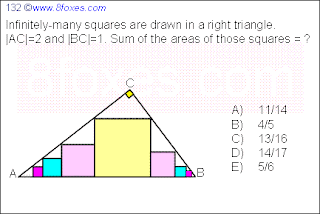## Wednesday, August 26, 2009

### Fox 1321.First we watch the biggest square in the middle.

The three triangles around this square are similar.
Call the side of the square DEFG, with DG on AB, E on AC and F on BC.
Then: EC = 2/sqrt(5)*x and AE = sqrt(5)*x
AE+EC = AC = 2 so x = 2/7*sqrt(5) and area DEFG = x^2 = 20/49.

Now we watch any right-angled triangle similar to ABC,with a square in the right angle.
When the square had sides x, then it's easy to see that the two remaining triangles are similar with the original triangle, one with factor 2/3 and the other with factor 1/3.
And it follows that the side x = hypothenusa/(3/2*sqrt(5)) = 2/(3sqrt(5)) * hypothenusa.

Now we distinguish between the left and right side of the figure.

Right side:
In every step the triangle gets smaller with factor 1/3, so every square gets smaller with factor 1/3. The first square has side x0 = 2/(3sqrt(5))*BF = 2sqrt(5)/21 and area (x0)^2=20/441.
The areas of the squares on the right side give a geometric sequence, with area An = 20/441*(1/9)^n.
The total area of the squares on the right side is equal to Sum(n=0 to infinity)An = 20/441*9/8 = 5/98

Left side:
The first square has side 2/(3sqrt(5))*AE = 4sqrt(5)/21 and area 80/441.
The area give a geometric sequence: Bn = 80/441*(4/9)^n so the total area of the squares on the lift side is equal to 80/441*9/5 = 16/49

The total sum of the areas of all squares = 20/49 + 16/49 + 5/98 = 77/98 = 11/14

2.3.